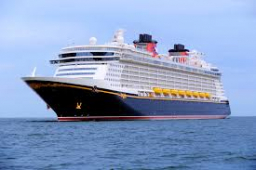# Boats 2

Three-quarters of the boats in the marina are white. 4/7 out of the remaining boats are blue, and the rest are red if there are nine red boats. How many boats are in the marina?

n =  84

### Step-by-step explanation:Did you find an error or inaccuracy? Feel free to write us. Thank you!

Tips for related online calculators
Need help calculating sum, simplifying, or multiplying fractions? Try our fraction calculator.
Do you have a linear equation or system of equations and looking for its solution? Or do you have a quadratic equation?